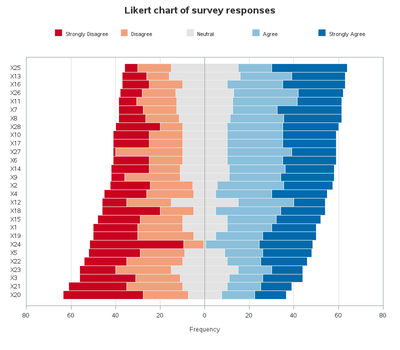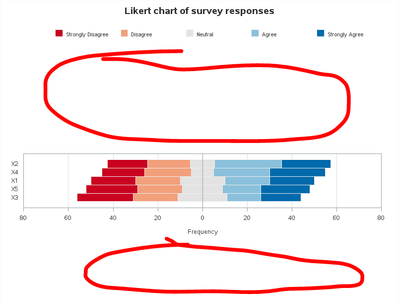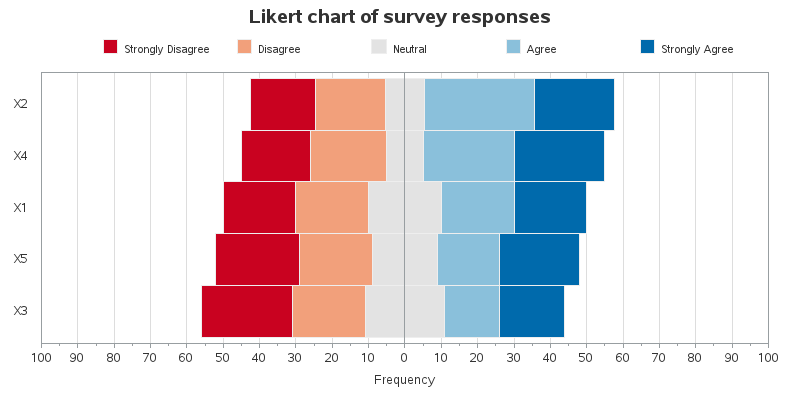## Probably easy to solve: error with proc gchart - Beginner

Hi, I struggle using an example code for my purpose...

For overview reasons, I attached the code below instead of at this place.

I found the example code on http://robslink.com/SAS/democd_compare/likert_info.htm

and it produces this output:Now I want to do a similar chart but only with 5 rows.

The problem is, that I dont know how to adjust the spacing then 😞

I just reduce the data to

``````data my_data;
length category \$3;
input category bin1 bin2 bin3 bin4 bin5;
sum=bin1+bin2+bin3+bin4+bin5;
datalines;
X1  20 20 20 20 20
X2  18 19 11 30 22
X3  25 20 22 15 18
X4  19 21 10 25 25
X5  23 20 18 17 22
run;``````

and I get a graphic with way too much spacing below and above the graph....Can somebody help me out? 🙂

Is there any way to add those?

I want to add 1 minortick between 2 ticks, so ticks for every 10 and grid for every step so -100 -90 -80 and so on.

I would do this normally with smth like

``minorgrid=4 minorticks=1 minortickcount=4``

but I dont know how I should add this. Many tries didnt worked and the sas documentation didnt helped me out. 😕

Thanks in advance to everyone who is trying to help me!

code:

``````%let name=likert;
filename odsout '.';

data my_data;
length category \$3;
input category bin1 bin2 bin3 bin4 bin5;
sum=bin1+bin2+bin3+bin4+bin5;
datalines;
X1  20 20 20 20 20
X2  18 19 11 30 22
X3  25 20 22 15 18
X4  19 21 10 25 25
X5  23 20 18 17 22
X6  16 15 20 25 24
X7  11 15 25 20 29
X8  12 15 23 24 26
X9   6 25 22 23 24
X10 16 15 20 25 24
X11  8 18 25 29 20
X12 11 20 30 25 14
X13 11 10 32 23 24
X14 17 14 22 25 22
X15 19 19 20 22 20
X16 12 15 20 25 28
X17 16 15 20 25 24
X18 26 15 10 29 20
X19 20 25 10 21 24
X20 36 20 15 15 14
X21 26 25 20 15 14
X22 19 25 20 15 21
X23 16 25 30 15 14
X24 42  9  1 24 24
X25  6 15 30 15 34
X26 10 15 26 29 20
X27  1 30 20 29 20
X28 20 10 20 25 25
run;

data plot_data; set my_data;
length hovertext \$300;
hovertext='title='||quote(
trim(left(category))||'0d'x||
'------------------------'||'0d'x||
trim(left(bin1))||' Strongly Disagree'||'0d'x||
trim(left(bin2))||' Disagree'||'0d'x||
trim(left(bin3))||' Neutral'||'0d'x||
trim(left(bin4))||' Agree'||'0d'x||
trim(left(bin5))||' Strongly Agree'
);
bin=3; value=bin1*-1; output;
bin=2; value=bin2*-1; output;
bin=1; value=(bin3/2)*-1; output;
bin=4; value=(bin3/2); output;
bin=5; value=bin4; output;
bin=6; value=bin5; output;
run;

proc format;
value bin_fmt
1="Neutral"
2="Disagree"
3="Strongly Disagree"
4=" Neutral"
5="Agree"
6="Strongly Agree"
;
run;

proc format; picture posval low-high='000,009'; run;

goptions device=png;
goptions xpixels=800 ypixels=700;
goptions noborder;

ODS LISTING CLOSE;
ODS HTML path=odsout body="&name..htm"
(title="Likert Survey Chart") style=htmlblue;

goptions gunit=pct ftitle='albany amt/bold' ftext='albany amt' htitle=14pt htext=10pt ctext=gray33;

legend1 label=none order=(3 2 1 5 6) value=(justify=left h=8pt)
position=(top center) across=5 shape=bar(.15in,.15in)
offset=(3,0);

pattern3 v=s c=cxc90220;
pattern2 v=s c=cxf2a07b;
pattern1 v=s c=cxe3e3e3;
pattern4 v=s c=cxe3e3e3;
pattern5 v=s c=cx8ac0db;
pattern6 v=s c=cx006aac;

axis1 label=('Frequency') order=(-80 to 80 by 20) minor=none offset=(0,0);

axis2 label=none offset=(3,3);

title1 ls=1.5 "Likert chart of survey responses";
title2 a=90 h=2 ' ';
title3 a=-90 h=2 ' ';

proc gchart data=plot_data;
format bin bin_fmt.;
format value posval.;
hbar category / discrete
type=sum sumvar=value nostats descending
subgroup=bin legend=legend1
raxis=axis1 maxis=axis2
space=0 width=1.3
coutline=grayee
autoref cref=graydd clipref
html=hovertext
des='' name="&name";
run;

quit;
ODS HTML CLOSE;
ODS LISTING;``````

1 ACCEPTED SOLUTION

Accepted Solutions

## Re: Probably easy to solve: error with proc gchart - Beginner

Since you have fewer bars, you'll want to increase the width= value to increase the width of the bars, to help fill the space. I increased to width=7

And now that the bars are wider, you'll need to increase the midpoint axis (maxis) offset, to give the wider bars more space (otherwise they'll extend outside the axes). I used axis2 offset=(8,8).

For only 5 bars, you probably don't want the graph to be as tall as the example containing many more bars, therefore I reduced the size using goptions ypixels=500

And to get one minor tick mark, I changed minor=none to minor=(number=1)

Now that you know which settings to adjust, you can adjust them to your liking. Here's my modified version of the code, using your data with 5 bars, and an image of what the output looks like:

``````data my_data;
length category \$3;
input category bin1 bin2 bin3 bin4 bin5;
sum=bin1+bin2+bin3+bin4+bin5;
datalines;
X1  20 20 20 20 20
X2  18 19 11 30 22
X3  25 20 22 15 18
X4  19 21 10 25 25
X5  23 20 18 17 22
run;

data plot_data; set my_data;
length hovertext \$300;
hovertext='title='||quote(
trim(left(category))||'0d'x||
'------------------------'||'0d'x||
trim(left(bin1))||' Strongly Disagree'||'0d'x||
trim(left(bin2))||' Disagree'||'0d'x||
trim(left(bin3))||' Neutral'||'0d'x||
trim(left(bin4))||' Agree'||'0d'x||
trim(left(bin5))||' Strongly Agree'
);
bin=3; value=bin1*-1; output;
bin=2; value=bin2*-1; output;
bin=1; value=(bin3/2)*-1; output;
bin=4; value=(bin3/2); output;
bin=5; value=bin4; output;
bin=6; value=bin5; output;
run;

proc format;
value bin_fmt
1="Neutral"
2="Disagree"
3="Strongly Disagree"
4=" Neutral"
5="Agree"
6="Strongly Agree"
;
run;

proc format; picture posval low-high='000,009'; run;

goptions device=png;
goptions xpixels=800 ypixels=400;
goptions noborder;

ODS LISTING CLOSE;
ODS HTML path=odsout body="&name..htm"
(title="Likert Survey Chart") style=htmlblue;

goptions gunit=pct ftitle='albany amt/bold' ftext='albany amt' htitle=14pt htext=10pt ctext=gray33;

legend1 label=none order=(3 2 1 5 6) value=(justify=left h=8pt)
position=(top center) across=5 shape=bar(.15in,.15in)
offset=(3,0);

pattern3 v=s c=cxc90220;
pattern2 v=s c=cxf2a07b;
pattern1 v=s c=cxe3e3e3;
pattern4 v=s c=cxe3e3e3;
pattern5 v=s c=cx8ac0db;
pattern6 v=s c=cx006aac;

axis1 label=('Frequency') order=(-100 to 100 by 10) minor=(number=1) offset=(0,0);

axis2 label=none offset=(8,8);

title1 ls=1.5 "Likert chart of survey responses";
title2 a=90 h=2 ' ';
title3 a=-90 h=2 ' ';

proc gchart data=plot_data;
format bin bin_fmt.;
format value posval.;
hbar category / discrete
type=sum sumvar=value nostats descending
subgroup=bin legend=legend1
raxis=axis1 maxis=axis2
space=0 width=7
coutline=grayee
autoref cref=graydd clipref
html=hovertext;

``````6 REPLIES 6DanH_sas
SAS Super FREQ

## Re: Probably easy to solve: error with proc gchart - Beginner

If you drop the WIDTH option on the HBAR statement, does the space get reclaimed?

## Re: Probably easy to solve: error with proc gchart - Beginner

sadly no, the width option in the hbar is for the width of the bars for each "row", if I remove it, the "rows" are only closer together 😕DanH_sas
SAS Super FREQ

## Re: Probably easy to solve: error with proc gchart - Beginner

Do these three things:

1. Change the YPIXELS to 300 on the GOPTIONS statement.

2. Remove the OFFSET=(3,3) from the AXIS statement.

3. Remove the WIDTH option from the HBAR statement.

Let me know if that works for you.

## Re: Probably easy to solve: error with proc gchart - Beginner

Thanks for your advices, sadly it doesnt changed much. The 1. yielded to just shrinking of the bars, so one cant read the text anymore, but didnt changed any spacing.
The 2nd yielded to a smaller "overset" of the borders around the bar graphic, but didnt touched the spacings. And the 3rd yields only to that, that the bars are closer together 😕

## Re: Probably easy to solve: error with proc gchart - Beginner

Since you have fewer bars, you'll want to increase the width= value to increase the width of the bars, to help fill the space. I increased to width=7

And now that the bars are wider, you'll need to increase the midpoint axis (maxis) offset, to give the wider bars more space (otherwise they'll extend outside the axes). I used axis2 offset=(8,8).

For only 5 bars, you probably don't want the graph to be as tall as the example containing many more bars, therefore I reduced the size using goptions ypixels=500

And to get one minor tick mark, I changed minor=none to minor=(number=1)

Now that you know which settings to adjust, you can adjust them to your liking. Here's my modified version of the code, using your data with 5 bars, and an image of what the output looks like:

``````data my_data;
length category \$3;
input category bin1 bin2 bin3 bin4 bin5;
sum=bin1+bin2+bin3+bin4+bin5;
datalines;
X1  20 20 20 20 20
X2  18 19 11 30 22
X3  25 20 22 15 18
X4  19 21 10 25 25
X5  23 20 18 17 22
run;

data plot_data; set my_data;
length hovertext \$300;
hovertext='title='||quote(
trim(left(category))||'0d'x||
'------------------------'||'0d'x||
trim(left(bin1))||' Strongly Disagree'||'0d'x||
trim(left(bin2))||' Disagree'||'0d'x||
trim(left(bin3))||' Neutral'||'0d'x||
trim(left(bin4))||' Agree'||'0d'x||
trim(left(bin5))||' Strongly Agree'
);
bin=3; value=bin1*-1; output;
bin=2; value=bin2*-1; output;
bin=1; value=(bin3/2)*-1; output;
bin=4; value=(bin3/2); output;
bin=5; value=bin4; output;
bin=6; value=bin5; output;
run;

proc format;
value bin_fmt
1="Neutral"
2="Disagree"
3="Strongly Disagree"
4=" Neutral"
5="Agree"
6="Strongly Agree"
;
run;

proc format; picture posval low-high='000,009'; run;

goptions device=png;
goptions xpixels=800 ypixels=400;
goptions noborder;

ODS LISTING CLOSE;
ODS HTML path=odsout body="&name..htm"
(title="Likert Survey Chart") style=htmlblue;

goptions gunit=pct ftitle='albany amt/bold' ftext='albany amt' htitle=14pt htext=10pt ctext=gray33;

legend1 label=none order=(3 2 1 5 6) value=(justify=left h=8pt)
position=(top center) across=5 shape=bar(.15in,.15in)
offset=(3,0);

pattern3 v=s c=cxc90220;
pattern2 v=s c=cxf2a07b;
pattern1 v=s c=cxe3e3e3;
pattern4 v=s c=cxe3e3e3;
pattern5 v=s c=cx8ac0db;
pattern6 v=s c=cx006aac;

axis1 label=('Frequency') order=(-100 to 100 by 10) minor=(number=1) offset=(0,0);

axis2 label=none offset=(8,8);

title1 ls=1.5 "Likert chart of survey responses";
title2 a=90 h=2 ' ';
title3 a=-90 h=2 ' ';

proc gchart data=plot_data;
format bin bin_fmt.;
format value posval.;
hbar category / discrete
type=sum sumvar=value nostats descending
subgroup=bin legend=legend1
raxis=axis1 maxis=axis2
space=0 width=7
coutline=grayee
autoref cref=graydd clipref
html=hovertext;

``````## Re: Probably easy to solve: error with proc gchart - Beginner

perfect, thank you soo much 🙂
Discussion stats
• 6 replies
• 303 views
• 2 likes
• 3 in conversation